# I.E. Irodov Solutions on Radioactivity

Radioactivity is a phenomenon exhibited by a few matters of emitting energy and subatomic particles spontaneously. Radioactivity is due to the nuclear instability of an atom. This chapter deals with the properties of radioactivity of an atom. IE IRODOV Physics Solutions provides the solutions to various problems related to the concept. The questions are diversified covering all types and aid in understanding the basic fundamentals of the concept easily.

The solutions are based on the specific formulae, equations for every question. They help the students understand the topic and connect the theoretical and practical problems. Solving these kinds of problems helps to have a grip over the chapter. This makes the student confident and gives a good practice for the upcoming board and competitive examination. Solving numerous problems and examples enable the students to develop an interest in the subject and it automatically motivates them to aim for higher grades.

### I.E. Irodov Solutions on Radioactivity

1. Knowing the decay constant λ of a nucleus, find:

(a) the probability of decay of the nucleus during the time from 0 to t;

(b) the mean lifetime ζ of the nucleus.

Solution:

1. a) The probability of survival (i.e. not decaying) in time t is e-λt. Hence the probability of decay is 1 - e-λt

b) The probability that the particle decays in time dt around time t is the difference

e-λt - e-λ(t+dt) = e-λt [1 - e-λt dt ] = λe-λt dt

Therefore, the mean life-time is

$T= \int_o^\infty t \lambda e^{- \lambda t} dt/\int_o^\infty \lambda e^{- \lambda t} dt$

= 1/λ

2. What fraction of the radioactive cobalt nuclei whose halflife is 71.3 days decays during a month?

Solution:

1. At first, we will calculate λ

$\lambda =\frac{\ln 2}{T_{\frac{1}{2}}}=9.722\times 10^{-3}$ per day

Hence fraction decaying in a month = 1-eλt= 0.253

3. How many beta-particles are emitted during one hour by 1.0μg of Na24 radionuclide whose half-life is 15 hours?

Solution:

1. Here

$N_{0} =\frac{1\mu g}{24 g}\times 6.023\times 10^{23}= 2.51\times 10^{16}$

Also,$\lambda =\frac{\ln 2}{T_{\frac{1}{2}}}=0.04621$ per hour

So the number of β rays emitted in one hour is No (1 - e-λt) = 1.13 x1015

4. To investigate the beta-decay of Mg23 radionuclide, a counter was activated at the moment t = 0. It registered N1 beta-particles by a moment t1 = 2.0s, and by a moment t2 = 3t1 the number of registered beta-particles was 2.66 times greater. Find the mean lifetime of the given nuclei.

Solution:

1. If N0 is the number of radio nuclei present initially, then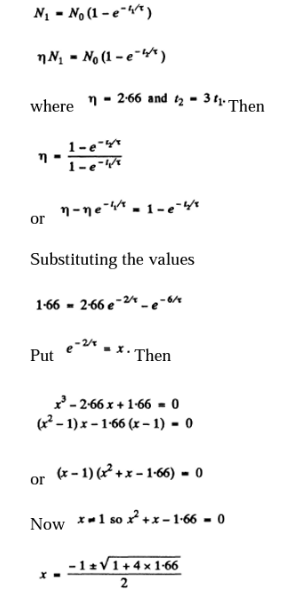The negative sign has to be rejected as x >0.

Thus x = 0.882

$\tau =\frac{-2}{ln 0.882}= 15.9 S$

5. The activity of a certain preparation decreases 2.5 times after 7.0 days. Find its half-life.

Solution:

1. If the half-life is T days

$(2)^{\frac{-7}{T}}=\frac{1}{2.5}$

Hence,

${\frac{7}{T}}=\frac{\ln 2.5}{\ln2}$

$T=\frac{7\ln 2}{\ln 2.5}=5.30 days$

6. At the initial moment, the activity of a certain radionuclide totalled 650 particles per minute. What will be the activity of the preparation after half its half-life period?

Solution:

1. The activity is proportional to the number of parent nuclei (assuming that the daughter is not radioactive). In half its half-life period, the number of parent nuclei decreases by a factor.

$2^{\frac{-1}{2}}=\frac{1}{\sqrt{2}}$

So activity decreases to $\frac{650}{\sqrt{2}}=450$ articles per minute.

7. Find the decay constant and the mean lifetime of Co55 radionuclide if its activity is known to decrease 4.0% per hour. The decay product is nonradioactive.

Solution:

1. If the decay constant (in (hour)-1 ) is λ., then the activity after one hour will decrease by a factor e~λ

Hence 0.96 = e~λ

λ = 1.11 X 10-5 S-1= 0.0408 per hour

The mean lifetime is 24.5 hour

8. A U238 preparation of mass 1.0 g emits 1.24 X104 alpha particles per second. Find the half-life of this nuclide and the activity of the preparation.

Solution:

1. Here

$N_{o}= \frac{1}{238}\times 6.023 \times 10^{23}$

= 2.531 X 1021

The activity is A = 1.24 x 104 dis/sec

$\lambda =\frac{A}{N_{0}}= 4.90\times 10^{18} per second$

Hence the half life is

$T_{\frac{1}{2}}=\frac{\ln 2}{\lambda }=4.49\times 10^{18} per second$

9. Determine the age of ancient wooden items if it is known that the specific activity of C14 nuclide in them amounts to 3/5 of that in lately felled trees. The half-life of C14 nuclei is 5570 years.

Solution:

1. In old wooden atoms, the number of C14 nuclei steadily decreases because of radioactive decay. (In live trees biological processes keep replenishing C14 nuclei maintaining a balance. This balance starts getting disrupted as soon as the tree is felled.)

If T1/2 is the half life of C14 then

$e^{-t \frac{\ln 2}{T_{\frac{1}{2}}}}=\frac{3}{5}$

Hence, $t= T_{\frac{1}{2}}\frac{\ln\frac{5}{3}}{\ln 2}= 4105$ years≅ 4.1 X 103 years

10. In a uranium ore the ratio of U238 nuclei to Pb206 nuclei is η = 2.8. Evaluate the age of the ore, assuming all the lead Pb206 to be a final decay product of the uranium series. The half-life of U238 nuclei is 4.5.109 years.

Solution:

1. What this implies is that in the time since the ore was formed $\frac{\eta }{1+\eta } U^{238}$ nuclei have remained undecayed. Thus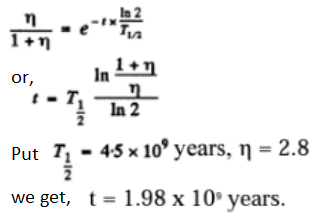11. Calculate the specific activities of Na24 and U235 nuclides whose half-lifes are 15 hours and 7.1 x 108 years respectively.

Solution:

1. The specific activity of Na24 is

$\lambda \frac{N_{A}}{M}=\frac{N_{A}\ln 2}{MT\frac{1}{2}}= 3.22\times 10^{17} dis/ (g.S)$

Here M = molar weight of Na24= 24 g NA is Avogadro number & T1/2 is the half-life of Na24 Similarly the specific activity of U235 is

\frac{6.023\times10^{23}\times\ln 2}{235 \times\10^{8}\times 365\times86400}

= 0.793 x 105dis/ (g.S)

12. A small amount of solution containing Na24 radionuclide with activity A = 2.0 x 103disintegrations per second was injected in the bloodstream of a man. The activity of 1 cm3of blood sample taken t = 5.0 hours later turned out to be A' = 16 disintegrations per minute per cm3. The half-life of the radionuclide is T = 15 hours. Find the volume of the man's blood.

Solution:

1. Let V = volume of blood in the body of the human being. Then the total activity of the blood is A' V. Assuming all this activity is due to the injected Na24 and taking account of the decay of this radionuclide, we get

VA’ = A e-λt

Now, λ = (ln 2)/15 per hour and t = 5 hour

Therefore, V = (A/A’) e- ln(⅔) = [2.0 x 103]/[16/60] x e -ln(⅔)cc = 5.99 litre

13. The specific activity of a preparation consisting of radioactive Co58 and nonradioactive Co59 is equal to 2.2 x1012 dis/(s•g). The half-life of Co58 is 71.3 days. Find the ratio of the mass of radioactive cobalt in that preparation to the total mass of the preparation (in percent)

Solution:

1. We see that Specific activity of the sample

=1/[M+M’]

(Activity of M gm of Co58 in the sample)

Here M and At are the masses of Cobalt 58 and Cobalt 59 in the sample. Now activity of M gm of Co58.

= M/58 x 6.023 x 1023 x ln 2/[71.3 x 86400] dis/sec

From the given data,

1.168 x 1015[M/(M+M’)] = 2.2 x 1012

Or M/(M+M’) = 1.88 x 10-3.

14. A certain preparation includes two beta-active components with different half-lifes. The measurements resulted in the following dependence of the natural logarithm of preparation activity on time t expressed in hours:

 t 0 1 2 3 5 7 20 14 20 ln A 4.1 3.6 3.1 2.6 2.06 1.82 1.6 1.32 0.9

Find the half-lifes of both components and the ratio of radioactive nuclei of these components at the moment t = 0.

Solution:

1. Suppose N1 N2 are the initial number of component nuclei whose decay constants are λ1 , λ2 ( in (hour)-1 Then the activity at any instant is

$A= \lambda _{1}N_{1}e^{-\lambda _{1}t} + \lambda _{2}N_{2}e^{-\lambda _{2}t}$

The activity so defined is in units dis/hour. We assume that data In A given is of its natural logarithm. The daughter nuclei are assumed nonradioactive.

We see from the data that at large t the change in In A per hour of elapsed time is constant and equal to - 0.07.

Thus λ2 = 0.07 per hour We can then see that the best fit to data is obtained by

$A(t)= 51.1e^{-0.661} + 10. 0 e^{-0.07 t}$

[To get the fit we calculate We see that A(t)=e0.07 t it reaches the constant value 10.0 at t = 7, 10, 14, 20 very nearly. This fixes the second term. The first term is then obtained by subtracting out the constant value 10.0 from each value of A(t)=e0.07 t in the data for small t ]

Thus we get λ1 = 0.66 per hour

T1 = 1.05 hour and T2 = 9.9 hours [half-lives]

Ratio: N1/N2 = [51.1/10.0] x λ2/ λ1 = 0.54

15. A P32 radionuclide with half-life T = 14.3 days is produced in a reactor at a constant rate q = 2.7x109 nuclei per second. How soon after the beginning of production of that radionuclide will its activity be equal to A = 1.0 x 109 dis/s?

Solution:

1. Production of the nucleus is governed by the equation

dN/dt = g - λN

Where g -> supply and λN -> decay

We observe that N will approach a constant value g/λ. This can also be proved directly. Multiply by and write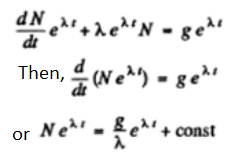At t = 0, when the production is started, N=0

so, 0 = g/λ + constant

Hence, N = (g/λ) (1 - e-λt)

Now the activity is A = λN = g(1 - e-λt)

Also from the problem, we know that

1/2.7 = 1 - e-λt

This gives λt = 0.463

So, t = [0.463xT)/0.693 = 9.5 days

Algebraically, t = -T/(ln 2) ln (1 - A/g)

16. A radionuclide A1 with decay constant λ1 transforms into a radionuclide A2 with decay constant λ2. Assuming that at the initial moment the preparation contained only the radionuclide A1, find:

(a) the equation describing accumulation of the radionuclide A2 With time;

(b) the time interval after which the activity of radionuclide A2 reaches the maximum value.

Solution:

1. (a) Suppose N1 and N2 are the number of two radionuclides A1, A2 at time t. Then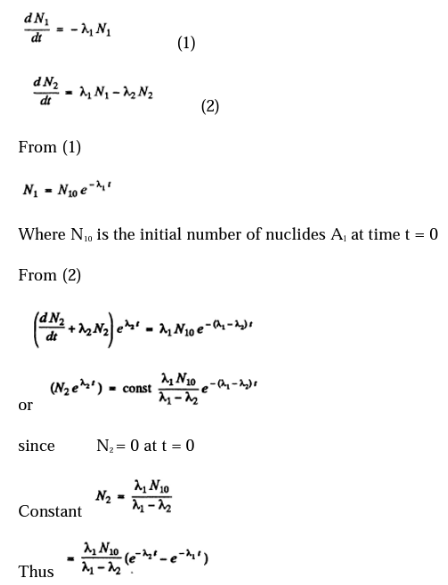(b) The activity of nuclide A2 is λ2 N2. This is maximum when N2 is maximum. That happens when

dN2/dt = 0

This requires

λ2 e-λ_2 t_m = λ1 e-λ_1 t_m

tm = [ln(λ12)/(λ12)

17. Solve the foregoing problem if λ1 = X 2 = X.

Solution:

1. (a)This case can be obtained from the previous one by putting

$\lambda _{2}-\lambda _{1}=\varepsilon$

Where ε is very small and ε ->0 at the end. Then

$N_2 = \frac{\lambda_1 N_{10}}{\epsilon} (e^{\epsilon t} - 1)e^{-\lambda_1 t}= \lambda_1 t e^{-\lambda_1 t} N_{10}$

Or dropping the subscript at 1 as the two values are equal, we get

$N_2 = N_{10}\lambda t e^{-\lambda t}$

(b) This is maximum when

$\frac{dN_{2}}{dt}=0$

$t=\frac{1}{\lambda }$

18. A radionuclide A1 goes through the transformation chain A1 → A2 → A3 (stable) with respective decay constants λ1 and λ2. Assuming that at the initial moment the preparation contained only the radionuclide A1 equal in quantity to N10 nuclei, find the equation describing accumulation of the stable isotope A3.

Solution:

1. Here we have the equations

dN1/dt = -λ1N1 and dN2/dt = -λ2N2 and dN3/dt = -λ3N3

we from previous problem, N1 = N10 e-λ_1t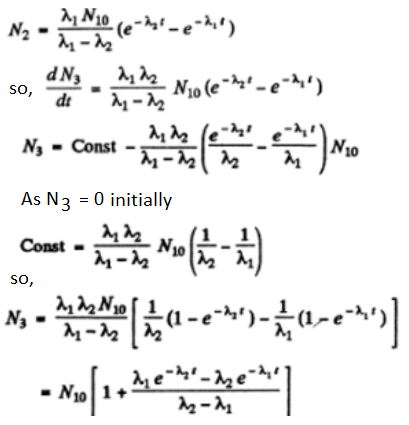19. A Bi210 radionuclide decays via the chain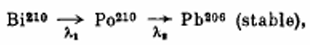where the decay constants are λ1= 1.60 X 10-6S-1, λ2= 5.80x10-8 s-1. Calculate alpha- and beta-activities of the Bi210 preparation of mass 1.00 mg a month after its manufacture.

Solution:

1. We have the chain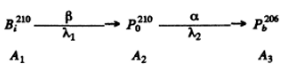of the previous problem initially

N_{10}=\frac{10^{-3}}{210}\times\6.023\10^{23}=2.87\times 10^{18}

A month after preparation

N1 = 4.54 x 1016

N2 = 2.52 x 1018 using the results of the previous problem.

Then

Aβ = λ1N1 = 0.725 x 1011 dis/sec

Aα = λ2N2 = 1.46 x 1011 dis/sec

20. (a) What isotope is produced from the alpha-radioactive Ra228 as a result of five alpha-disintegrations and four β-disintegrations?

(b) How many alpha- and β-decays does U 238 experience before turning finally into the stable Pb206 isotope?

Solution:

1. (a) Ra has Z - 88, A - 226 After 3 a emission and 4 p (electron) emission

A - 206

Z - 88 + 4-5x2 - 82

The product is 82Pb206

(b) We require,

-ΔZ = 10 = 2n - m

-ΔA = 32 = ft x 4

Here n = no. of α emissions

m = no. of β emissions

Thus n = 8, m = 6